Simultaneous Covariance Driven Correspondence (CDC) and Transformation Estimation in the Expectation Maximization Framework

This page gives a high level overview of our research on Covariance Driven Correspondences (CDC). For more details, please refer to our article published in CVPR 2007 proceedings.

Contents

Overview

• Novel registration algorithm, that depends fundamentally on the estimation of uncertainty in point correspondences
• Uncertainty derived from the covariance matrices of the individual point locations and from the covariance matrix of the estimated transformation parameters
• Robust objective function and an EM-like algorithm to simultaneously estimate the transformation parameters, their covariance matrix, and the likely correspondences
• No annealing schedule nor an explicit outlier process needed
• Broader domain of convergence than the Iterative Closest Point (ICP) algorithm and is more robust to missing or extraneous structures in the data than Robust Point Matching (RPM) 

Introduction

Iterative closest point (ICP) algorithm starts from an initial estimate of the transformation parameters, uses this to generate an initial set of correspondences, re-estimates the parameters, and iterates.

Problem of standard ICP (illustrated in Figure 1)

• for moving points (red circles) along the horizontal line of the 'H', the closest fixed points (blue crosses) are along one of the two vertical lines
• noise in the locations and surface normals of points along the sides of the 'H' produces constraints that resist changes in the transformation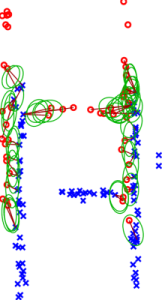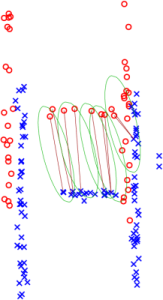Robust Point Matching Algorithm (RPM)  starts by initially considering all possible matches, and then, using an annealing schedule, gradually moves toward unique correspondences as the transformation is refined.

Problem of RPM

• works poorly, when there are missing or extraneous structures (Figure 2(a))
• in the early stages of its computation RPM tends toward aligning the centers of mass of the points, producing a bias in the estimate when there are extraneous structures that can not be overcome in later stages of the computation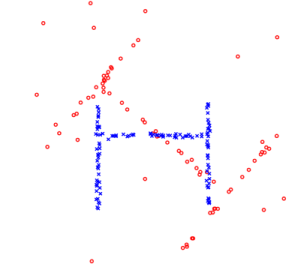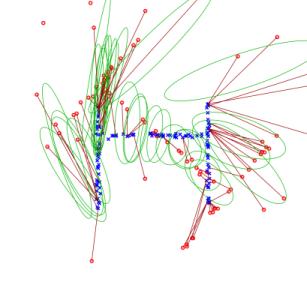CDC fixes the problems

• uncertainty in the transformation estimate produces uncertainty in the mapped point positions that is predominantly in the vertical direction which allows correspondences between points on the crossbars of the two H's to be preferentially established
• correspondences between points along the two sides of the 'H' indicate large uncertainty in the vertical direction so they do not work against the changes in the transformation

Covariance of the Alignment Error

Alignment Error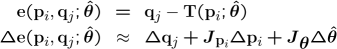Covariance of a Correspondence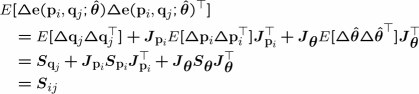Residual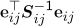Error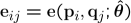Robust Objective Function

Distribution of Error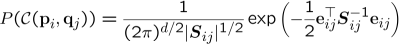Log Likelihood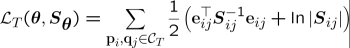Robust Function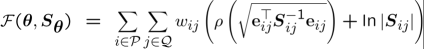where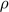......Constant Multiplied Beaton-Tukey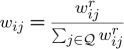......Competitive Weights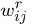......Robust Weights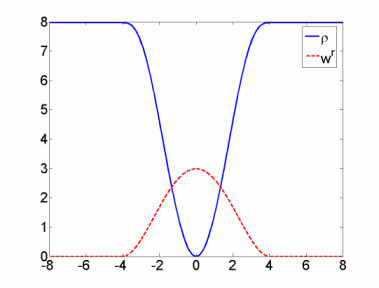Algorithm Outline

Starting from an initial transformation parameter vector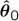, the point sets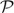and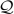, and the point covariances:

1. Compute an initial estimate of transformation parameter covariance matrix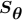.
2. Iterate until convergence
• (a) Recompute the weights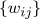for all correspondences keeping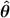andfixed.
• (b) Update the parameter estimateholdingandfixed.
• (c) Recompute the weightsas in Step 2(a).
• (d) Update the covariance matrix estimateholdingandfixed.

Results

Example of alignment of two H-like shapes:

1. Initial transformation and matches
2. Initial covariance estimate
3. Covariance estimate after a few iterations
4. Most uncertainty in vertical direction
5. Lower uncertainty of the parameter estimate
6. Fully aligned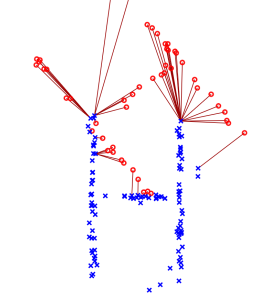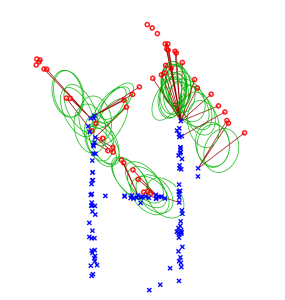(1) (2)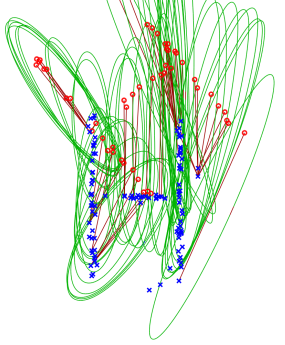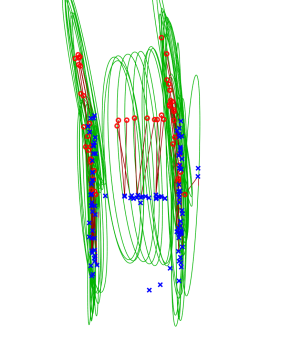(3) (4)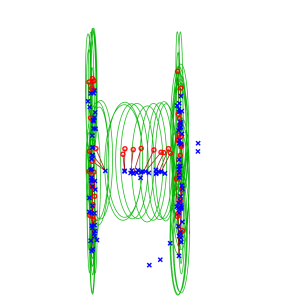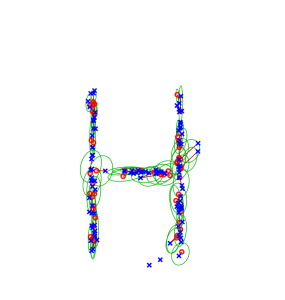(5) (6)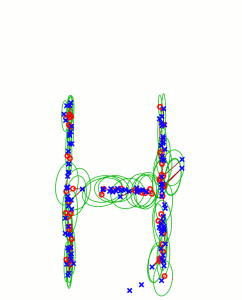Convergence analysis with different initializations

• moving shape (red circles) initialized at the marked locations around a circle with five different orientations
• green line segment indicates a location and orientation from which the algorithm converged correctly
• red segment indicates a failure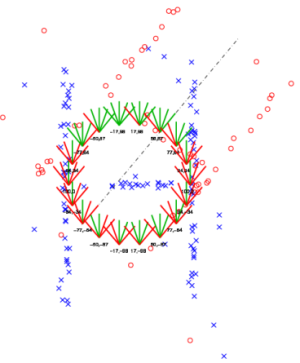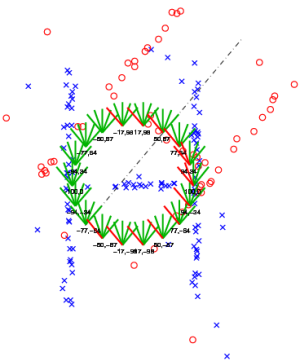(a) (b)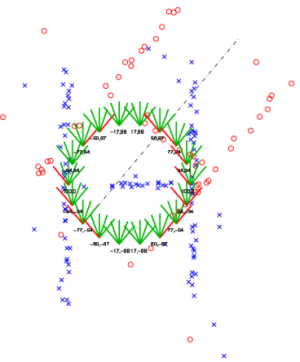(c)

The effect of noise and structural changes and the way RPM and CDC handles them.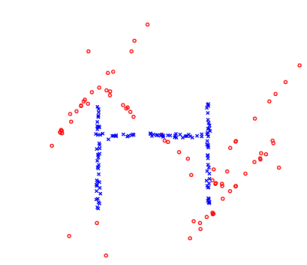(a) Noisy shapes (b) Structural changes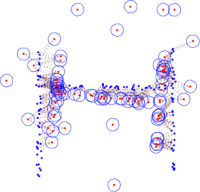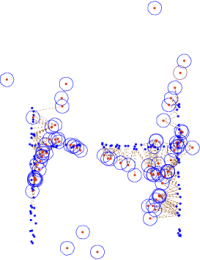(c) RPM alignment (d) RPM alignment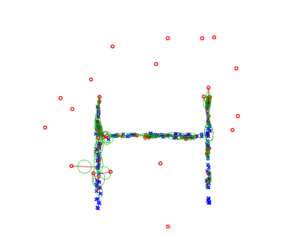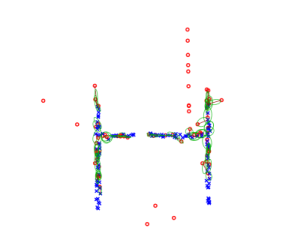(e) CDC alignment (f) CDC alignment

Dual-Bootstrap ICP Algorithm (DBICP) 

• DBICP
• (a) initial transforms accurate over a small region
• (b) ICP estimation, refinement and region growth
• (c) sophisticated set of decision criteria
• 81% of initial estimates refined to an accurate transform
• Step (b) based on CDC: correct alignment of 36% of the initializations that failed when using ICP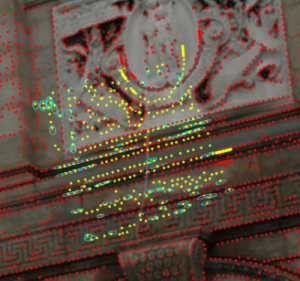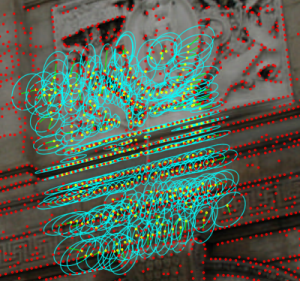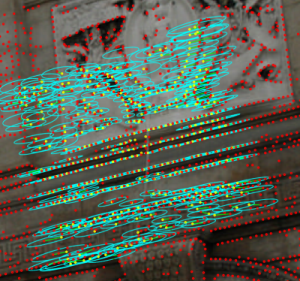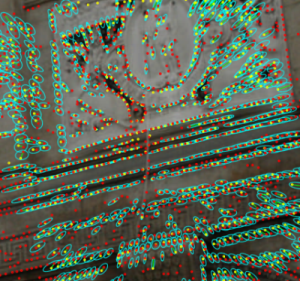3D Rigid Registration

• Align scans with different viewpoints and pairs with different overlaps (more difficult than prerotating data to register against itself)
• Initialize with identity transform
• ICP aligned 9 scan pairs
• CDC aligned 16, including the 9 ICP aligned
• CDC failed on only two pairs with inter-scan rotation angle less than 80 degrees (failures: 56 and 59 degree rotations)
• ICP failed on most pairs rotated by more than 45 degrees.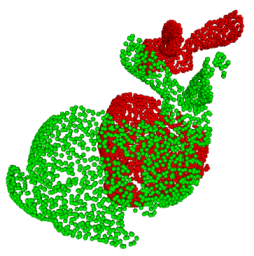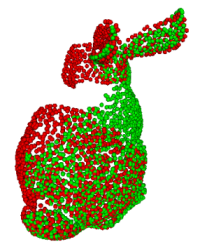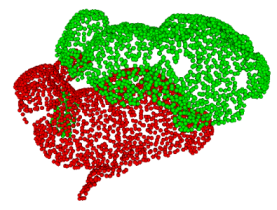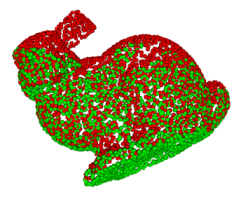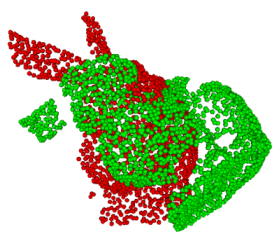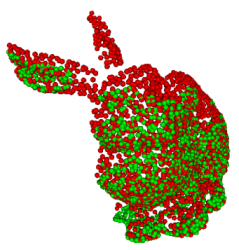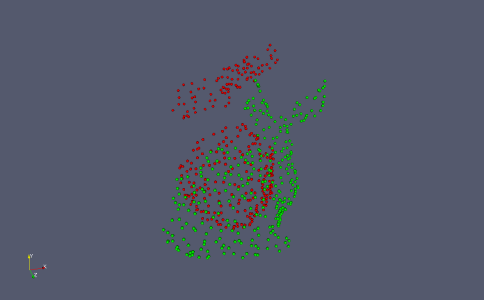Summary

• Uncertainty in correspondences drives matching and parameter estimation
• Parameter covariance estimated as a free variable
• Robust EM algorithm
• Broader domain of convergence than ICP, local characteristic of ICP removed
• Euclidean and normal distance ICP are special cases -> generalizing (replacing) ICP
• More robust to noise and missing or extraneous structures than RPM

1.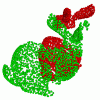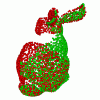Sofka, M., Yang, G., V. Stewart, C., 2007. Simultaneous Covariance Driven Correspondence (CDC) and Transformation Estimation in the Expectation Maximization. In: Proceedings of the IEEE Conference on Computer Vision and Pattern Recognition (CVPR). Minneapolis, MN, USA.